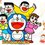# Discussion of SMO Senior Round 2 Qns 2014

The link to the Round 2 Paper for this year's Singapore Mathematical Olympiad is here. The Round 2 competition takes place just yesterday (28 June) so it's pretty new.

Here's the place to discuss the answers to these qns. :) So for those interested to try them or discuss them, feel free to do so!Note by Happy Melodies
6 years, 1 month ago

This discussion board is a place to discuss our Daily Challenges and the math and science related to those challenges. Explanations are more than just a solution — they should explain the steps and thinking strategies that you used to obtain the solution. Comments should further the discussion of math and science.

When posting on Brilliant:

• Use the emojis to react to an explanation, whether you're congratulating a job well done , or just really confused .
• Ask specific questions about the challenge or the steps in somebody's explanation. Well-posed questions can add a lot to the discussion, but posting "I don't understand!" doesn't help anyone.
• Try to contribute something new to the discussion, whether it is an extension, generalization or other idea related to the challenge.

MarkdownAppears as
*italics* or _italics_ italics
**bold** or __bold__ bold
- bulleted- list
• bulleted
• list
1. numbered2. list
1. numbered
2. list
Note: you must add a full line of space before and after lists for them to show up correctly
paragraph 1paragraph 2

paragraph 1

paragraph 2

[example link](https://brilliant.org)example link
> This is a quote
This is a quote
    # I indented these lines
# 4 spaces, and now they show
# up as a code block.

print "hello world"
# I indented these lines
# 4 spaces, and now they show
# up as a code block.

print "hello world"
MathAppears as
Remember to wrap math in $$ ... $$ or $ ... $ to ensure proper formatting.
2 \times 3 $2 \times 3$
2^{34} $2^{34}$
a_{i-1} $a_{i-1}$
\frac{2}{3} $\frac{2}{3}$
\sqrt{2} $\sqrt{2}$
\sum_{i=1}^3 $\sum_{i=1}^3$
\sin \theta $\sin \theta$
\boxed{123} $\boxed{123}$

Sort by:

I took the Junior paper though, and it was easier than I had thought previously, to be honest. I solved all 5 questions :)

- 6 years, 1 month ago

Good luck to all who took the SMO! Wish you all the best! :D

- 6 years, 1 month ago

5 was pretty obvious though... For 2 I used another method.

- 6 years, 1 month ago

Wut! For 4 you have to prove that a,b and c HAVE to be equal man.

- 6 years, 1 month ago

Okay okay fine. I'm removing that with all this debate going on... D:

- 6 years, 1 month ago

- 6 years, 1 month ago

Q3 just spam lah hahah

- 6 years, 1 month ago

How does Question 4 pop out without much effort at all

- 6 years, 1 month ago

Its the hardest question

- 6 years, 1 month ago

Answer to second is $(0,0,00$ and $(4,4,4)$

- 6 years, 1 month ago

Question asked for only positive reals.

- 6 years, 1 month ago

Sorry, Then only $(4,4,4)$

- 6 years, 1 month ago

Whats the proof?

- 6 years, 1 month ago

There is also a solution with AM-GM i think

- 6 years, 1 month ago

An easy way is to substitute $x=\sqrt{a},y=\sqrt{b},z=\sqrt{c}$ . The you get 3 equations. Try to find ab expression involving just one variable. So, we get $x\left( x-2\right)\left(x^{12}-3x^{11}+6x^{10}-14x^{9}+22x^{8}-28x^{7}+37x^{6}-35x^{5}+26x^{4}-21x^{3}+14x^{2}-12x+8\right) =0$. . Now this gives $x=2,x=0$. So, we get $a=4,0$. So, leaving $0$, we get the desired result

- 6 years, 1 month ago

right... and how did you prove that the huge polynomial has no positive real roots?

- 6 years, 1 month ago

Pure evil.

- 6 years, 1 month ago

Something like WLOG $a \geq b \geq c > 0$. Then $a+b \geq a+c$, yet $a\sqrt{b} \geq b\sqrt{c}$, which implies $a+c \geq a+b$ instead, so $b = c$. Similarly, $a = b = c$. And we are done.

- 6 years, 1 month ago

I think that's it @Victor Loh did you do that to?

- 6 years, 1 month ago

If anyone requires, the SMO Junior Round 2 2014 Qns can be found here

- 6 years, 1 month ago

Still weak at solving symmetric systems... Number 2....

- 6 years, 1 month ago